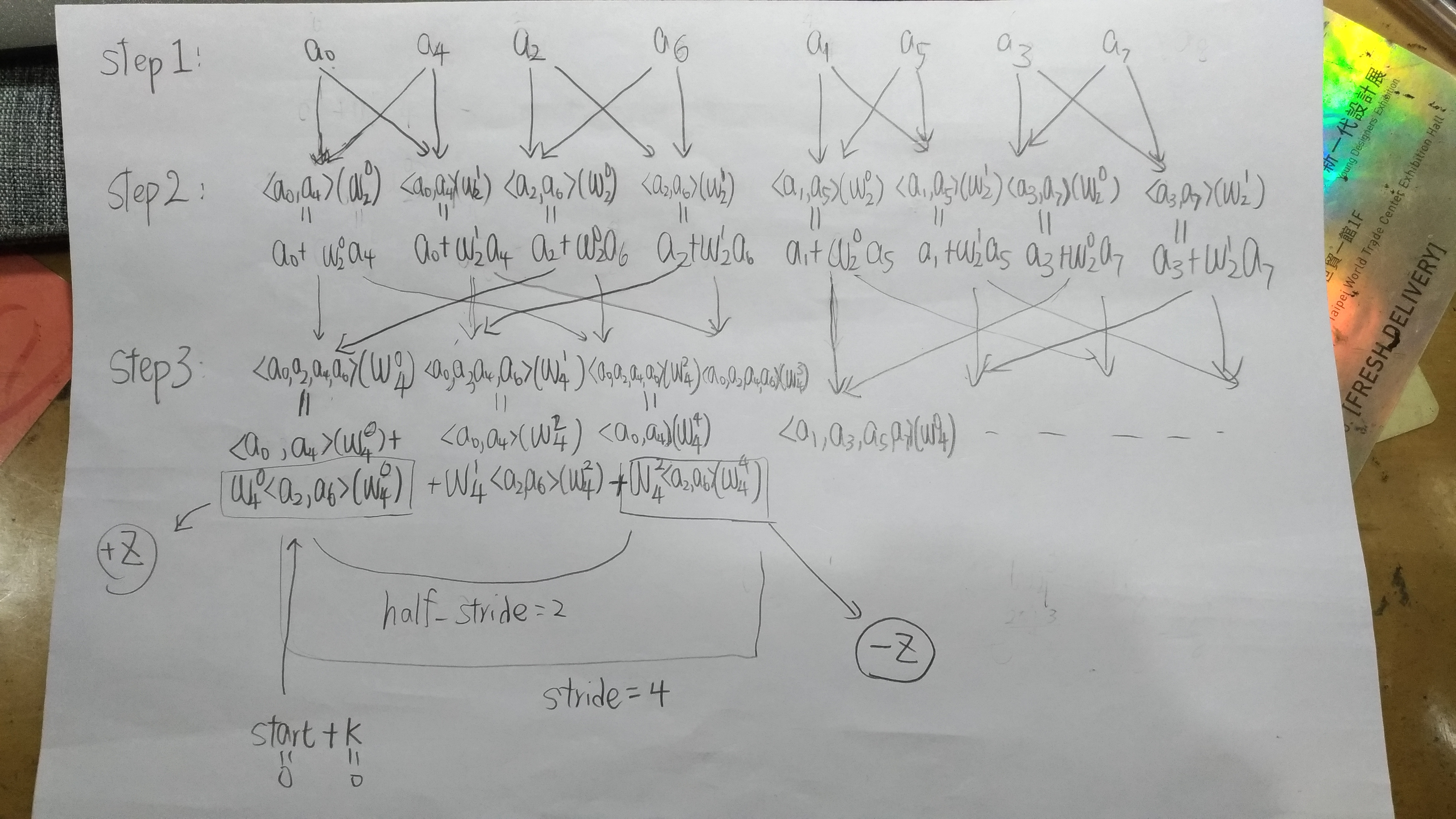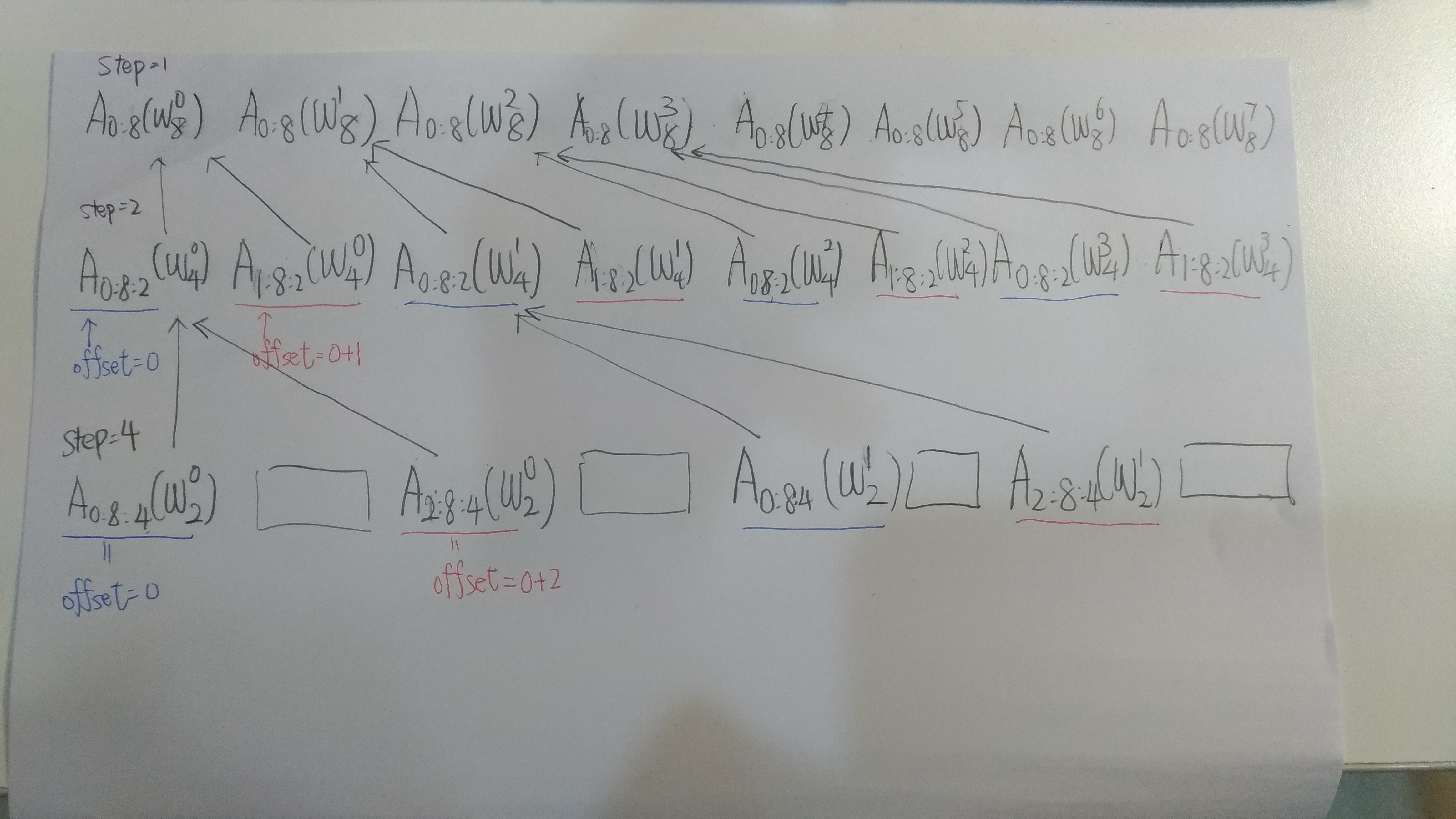Recently, I saw this codeforce problem https://codeforces.com/problemset/problem/1257/G, so I start to figure out how FFT works.

# Iterative FFT

Let $a$ be the original sequence and $b$ is the sequence after divide and conquer,

Then we can observe that:

Let’s initialize $j = 0$ index for $a$ and $i = 0$ index for $b$, note that we can accelerate the procedure by incremental and in-place fashion, that is

Given the fact that:

, we can actually exploit the previous step $j == reverse(i)$ result.

Let us rewrite it to: $reverse(j) == i$, then we can plug $j$ into the update step.

Though $reverse(reverse(j) + 1))$ seems crazy, it can actually be implemented fast by bitwise operations in reverse_add below.

Horay! Let’s see the code

int reverse_add(int x, int bit_length)
{
// try to find the most first bit that is 0
for(int l = 1 << bit_length; (x ^= l) < l; l >>= 1);
return x;
}

void bit_reverse(int n, complex_t *x)
{
int bit_length = (int) log2(n);
for(int i = 0, j = 0; i != n; ++i)
{
if(i > j) swap(x[i], x[j]);
}
}


Let us try this example:

where $w^{k}_{n} = e^{\frac{2 \pi i k}{n}}$.

And a polynomial ( I use the vector notation introduced by MIT open course):

Let’s see the computational graph:Code:

void transform(int n, complex_t *x, complex_t *w)
{
// Prepare the leaf nodes ( you can see this as a bottom-up approach )
bit_reverse(n, x);
for(int stride = 2; stride <= n; stride <<= 1)
{

int half_stride = stride >> 1;
// the start index of a stride region
for(int start = 0; start < n; start += stride)
{
// this loop will finish up [start, start + stride] region
for(int k = 0; k < half_stride; ++k)
{
complex_t z = x[start + half_stride + k] * w[n / stride * k];
// Use the phase property (see below)
x[start + half_stride + k] = x[start + k] - z;
x[start + k] = x[start + k] + z;
}
}
}
}


Note, in the code,

and the phase property:

# Recursively FFT

Here is the pseudocode:

fft(<a0, a1, a2, a3 ... , an-1>){
result = [None] * n
even = fft(<a0, a2, ... an-2>) // even[i] == A(w^{i}_{n/2})
assert(len(even) == n/2)
odd = fft(<a1, a3, ..., an-1>)
assert(len(odd) == n/2)

for(int i = 0; i < n; i++){
// A(w^i_n) = A_even(w^{2i}_n) + w^i_n * A_odd(w^{2i}_n)
// See the fact below.
result[i] = even[i % (n/2)] + w^i_n * odd[i % (n/2)]
}

return result
}


This is the code written by miskoo (See reference):

void fft(int n, complex<double>* buffer, int offset, int step, complex<double>* epsilon)
{
if(n == 1) return;
int m = n >> 1;
fft(m, buffer, offset, step << 1, epsilon);
fft(m, buffer, offset + step, step << 1, epsilon);
for(int k = 0; k != m; ++k)
{
int pos = 2 * step * k;
temp[k] = buffer[pos + offset] + epsilon[k * step] * buffer[pos + offset + step];
temp[k + m] = buffer[pos + offset] - epsilon[k * step] * buffer[pos + offset + step];
}

for(int i = 0; i != n; ++i)
buffer[i * step + offset] = temp[i];
}


For some people, this code is hard to understand at the first glance. So let us visualize it!:Note that for simplicity, I denote:

# Number Theoretic Transform (NTT)

## Procedure of NTT

• Let $n$ (must be the power of 2) be the transformed size of an input vector.
• Choose a prime number $p$ in the form of $p = k \cdot n + 1$, where $k \geq 1$. By Dirichilet prime theorem, you are guaranteed to find a $k$ such that $p$ is a prime.
• Let $g$ be the primitive $p - 1$ root of unitiy (or say, primitive root modulo $p$). (It is guaranteed that you can find a $g$ by the property of multiplicative group $\mathbb{Z}_p$, though I don’t know why.) Note that: $\{ g^0, g^1, g^2, ... g^{p-2} \}$ under modulo $p$ are unique.
• Define $w_n \equiv g^k \mod p$. By Euler theorem, $w^n_n = g^{kn} = g^{p-1} = g ^{\phi(p)}\equiv 1 \mod p$. Consider $w^{i}_n = g^{ik}$ for $% $, because $% $, by the property of Primitive root modulo n, we can make sure $\{ w^{0}_n, w^{1}_n, ... , w^{n-1}_n \}$ under modulo $p$ are unique. (You can prove that $w^{\frac{n}{2}}_{n} \equiv -1 \mod p$ because $\{ w^{0}_n, w^{1}_n, ... , w^{n-1}_n \}$ are unique. Therefore, every time you squre $\{ w^{0}_n, w^{1}_n, ... , w^{n-1}_n \}$, the size of this set will be divided by 2)

Note: If $w_n \equiv g^k \mod p$, then $w_{\frac{n}{2}} \equiv g^{2k} \mod p$ because $p = k \cdot n + 1 = 2k \cdot \frac{n}{2} + 1$.

## How to handle inverse of a finite field number

The last missing part is: how can we efficiently compute $w_n^{-1}$ (that is, the inverse of $g^k \mod p$)? In fact, we can use Extended Euclidean algorithm. Remember you can compute the $gcd(a, b)$ by

with initial value $r_{-2} = a$ and $r_{-1} = b$ (from Wikipedia).

Let us first see why we can definitely find the inverse of a number. Assume $gcd(a, b) = 1$, by Bezout’s identity, we are guaranteed to find integers $x, y$, such that:

Therefore, if we can calculate $x$, it will be the multiplicative inverse of $a$. In our case, $a$ is $g^k \mod p$ and $b$ is $p$ (which are coprime: $gcd(g^k \mod p, p) = 1$.

### Extended Euclidean algorithm

As the name shows, this is an extension of your high school Euclidean algorithm:

with $s_{-2} = 1, s_{-1} = 0, t_{-2} = 0, t_{-1} = 1$, terminate at $N$ step when $r_{N+1} = 0$, then $s = s_{N}$, $t = t_{N}$ will satisfy $s a + t b = gcd(a, b)$.

Proof: We claim that for each $j$ step,

Base case: $j == 0$

Therefore,

Assume $j \leq k - 1$, our claim is correct.

Induction step: by $k$’s step of Euclid algorithm:

Thus, at the $N$’s step (where $r_{N+1} = 0$), we will get

by the original definition of Euclid algorithm and our claim above.

### Alternative way to compute multiplicative inverse

In our case, our goal is to compute:

Nevertheless, in practice, we can just swap the result, because if the input polynomial is $A$ and:

Our goal is to get (By the definition of inverse FFT):

Recall that:

Observe that:

Therefore,

, which can be implemented by std::swap efficiently! (and no need to explicitly compute $w^{-i}_n$)

## How to handle negative number

(Thanks to yao11617’s contribution!) In NTT, we are actually perform addition, subtraction and multiplication over $GF(p)$ (a Finitie Field, where $p$ is a prime number). We can leverage the idea of 2’s complement. That is, we can represent the numbeer larger than $\frac{p-1}{2}$ as negative number.

Precisely, $F = \{0 == 0, 1 == 1, 2 == 2, ..., \frac{p-1}{2} == \frac{p-1}{2}, -\frac{p-1}{2} == \frac{p-1}{2} + 1, .... -1 == p-1\}$

Let us use several examples to make sure our idea is feasible:

Practically, you can map a negative number $a$ to $p + a$ and then restore it after performing NTT by $a - p$ (if the output coefficient is $a$)!

Isn’t it interesting?

# Practical Suggestion

• You can find the useful primitive root of unity table in FFT用到的各種素數 In case of that website crash, I backup that table here:
$p = r \cdot 2^{k} + 1$ (prime number) $r$ $k$ $g$ (primitive root)
3 1 1 2
5 1 2 2
17 1 4 3
97 3 5 5
193 3 6 5
257 1 8 3
7681 15 9 17
12289 3 12 11
40961 5 13 3
65537 1 16 3
786433 3 18 10
5767169 11 19 3
7340033 7 20 3
23068673 11 21 3
104857601 25 22 3
167772161 5 25 3
469762049 7 26 3
998244353 119 23 3
1004535809 479 21 3
2013265921 15 27 31
2281701377 17 27 3
3221225473 3 30 5
75161927681 35 31 3
77309411329 9 33 7
206158430209 3 36 22
2061584302081 15 37 7
2748779069441 5 39 3
6597069766657 3 41 5
39582418599937 9 42 5
79164837199873 9 43 5
263882790666241 15 44 7
1231453023109121 35 45 3
1337006139375617 19 46 3
3799912185593857 27 47 5
4222124650659841 15 48 19
7881299347898369 7 50 6
31525197391593473 7 52 3
180143985094819841 5 55 6
1945555039024054273 27 56 5
4179340454199820289 29 57 3